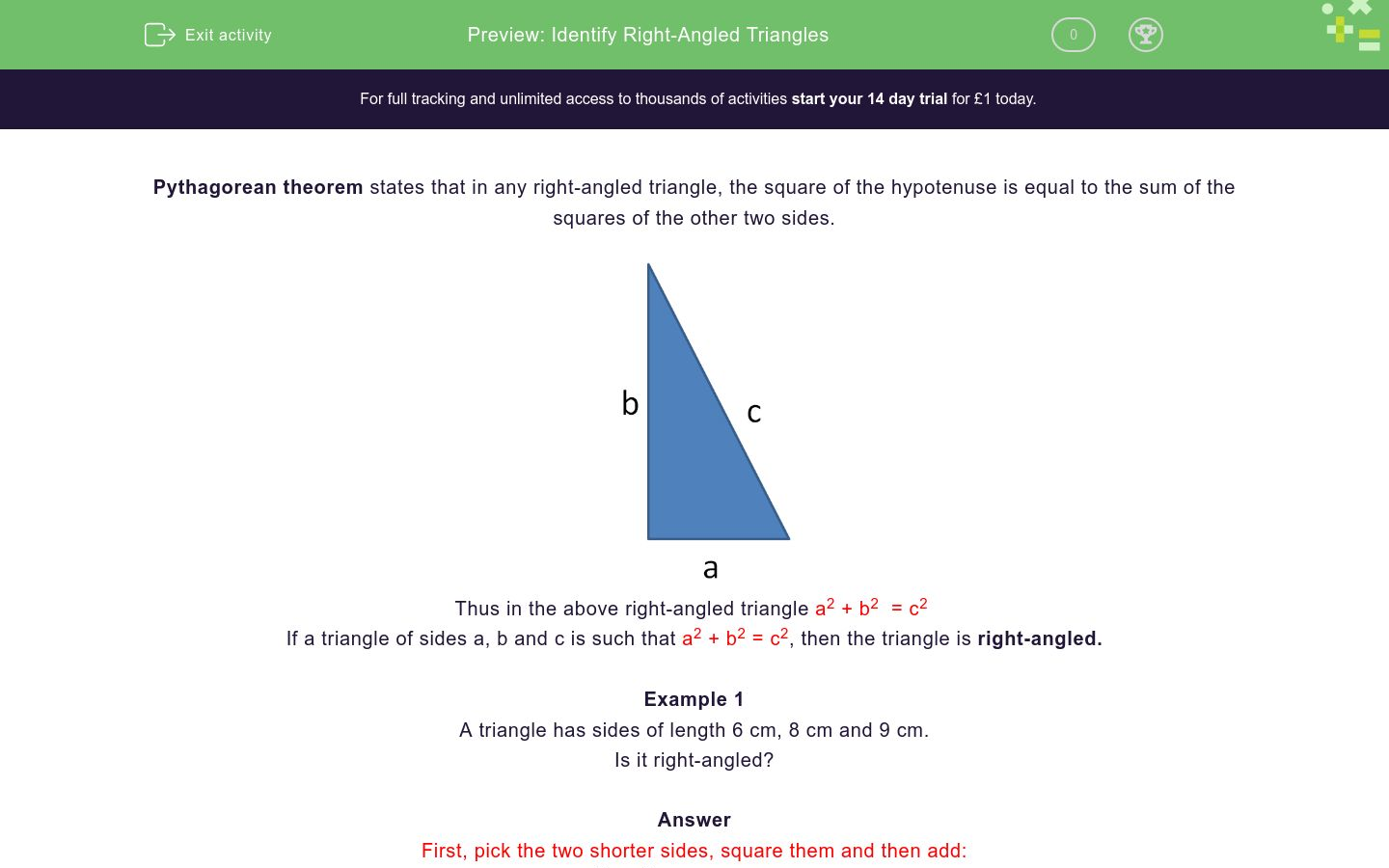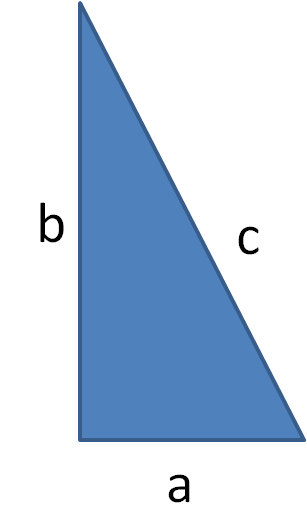# Is it a Right-Angled Triangle?

In this worksheet, students use the converse of Pythagoras' Theorem to determine whether or not a triangle is right-angled.Key stage:  KS 4

Curriculum topic:  Geometry and Measures

Difficulty level:### QUESTION 1 of 10

PYTHAGORAS' THEOREM

In any right-angled triangle, the square of the hypotenuse is equal to the sum of the squares of the other two sides.Thus in the above right-angled triangle, a2 + b2 = c2

CONVERSE

If a triangle of sides a, b and c is such that

a2 + b2 = c2

... then the triangle is right-angled.

Example 1

A triangle has sides of length 6 cm, 8 cm and 9 cm.

Is it right-angled?

Answer

First pick the two shorter sides, square them and add

62 + 82 = 36 + 64 = 100

Then square the longest side

92 = 81

So 62 + 82 ≠ 92 and the triangle is therefore not right-angled.

Example 2

A triangle has sides of length 5 cm, 13 cm and 12 cm.

Is it right-angled?

Answer

First pick the two shorter sides, square them and add

52 + 122 = 25 + 144 = 169

Then square the longest side

132 = 169

So 52 + 122 = 132 and the triangle is therefore right-angled.

A triangle has sides of length

3 cm, 4 cm and 5 cm.

Is it right-angled?

Yes

No

A triangle has sides of length

5 cm, 3 cm and 4 cm.

Is it right-angled?

Yes

No

A triangle has sides of length

10 cm, 13 cm and 12 cm.

Is it right-angled?

Yes

No

A triangle has sides of length

10 cm, 26 cm and 17 cm.

Is it right-angled?

Yes

No

A triangle has sides of length

10 cm, 26 cm and 24 cm.

Is it right-angled?

Yes

No

A triangle has sides of length

10 cm, 7 cm and 8 cm.

Is it right-angled?

Yes

No

A triangle has sides of length

10 cm, 7 cm and 16 cm.

Is it right-angled?

Yes

No

A triangle has sides of length

15 cm, 17 cm and 8 cm.

Is it right-angled?

Yes

No

A triangle has sides of length

45 cm, 37 cm and 11 cm.

Is it right-angled?

Yes

No

A triangle has sides of length

40 cm, 9 cm and 41 cm.

Is it right-angled?

Yes

No

• Question 1

A triangle has sides of length

3 cm, 4 cm and 5 cm.

Is it right-angled?

CORRECT ANSWER
Yes
• Question 2

A triangle has sides of length

5 cm, 3 cm and 4 cm.

Is it right-angled?

CORRECT ANSWER
Yes
• Question 3

A triangle has sides of length

10 cm, 13 cm and 12 cm.

Is it right-angled?

CORRECT ANSWER
No
• Question 4

A triangle has sides of length

10 cm, 26 cm and 17 cm.

Is it right-angled?

CORRECT ANSWER
No
• Question 5

A triangle has sides of length

10 cm, 26 cm and 24 cm.

Is it right-angled?

CORRECT ANSWER
Yes
• Question 6

A triangle has sides of length

10 cm, 7 cm and 8 cm.

Is it right-angled?

CORRECT ANSWER
No
• Question 7

A triangle has sides of length

10 cm, 7 cm and 16 cm.

Is it right-angled?

CORRECT ANSWER
No
• Question 8

A triangle has sides of length

15 cm, 17 cm and 8 cm.

Is it right-angled?

CORRECT ANSWER
Yes
• Question 9

A triangle has sides of length

45 cm, 37 cm and 11 cm.

Is it right-angled?

CORRECT ANSWER
No
• Question 10

A triangle has sides of length

40 cm, 9 cm and 41 cm.

Is it right-angled?

CORRECT ANSWER
Yes
---- OR ----

Sign up for a £1 trial so you can track and measure your child's progress on this activity.

### What is EdPlace?

We're your National Curriculum aligned online education content provider helping each child succeed in English, maths and science from year 1 to GCSE. With an EdPlace account you’ll be able to track and measure progress, helping each child achieve their best. We build confidence and attainment by personalising each child’s learning at a level that suits them.

Start your £1 trial